Methods for analyzing MTF in OpticStudio

Category: Product NewsMTF describes the transfer of modulation from the object to the image as a function of spatial frequency. It is commonly used to specify lens performance, and as an optimization and tolerancing target during lens design.

Modulation Transfer Function (MTF) is an important method of describing the performance of an optical system. As a consequence of applying Fourier theory to image-forming optical systems, MTF describes the contrast in the image of a spatial frequency presented in the scene being viewed.

MTF is measured per millimeter (mm) in focal space, and per milliradian (or some other angular unit) in afocal space. For sinusoidal targets, the MTF is measured in the sinusoidal response - or cycles. For bar targets, it is measured in line pairs. OpticStudio can compute the MTF for either target type.

In this blog post, we look at various sampling schemes and MTF algorithms available in OpticStudio, and how each of those algorithms can be individually incorporated into the Merit Function. While each algorithm has its corresponding optimization operand, the Contrast optimization method is preferred for optimizing against the MTF.

MTF example

The bitmap image analysis window below shows good image quality in the section showing the three boys: but how do we quantify "good"? The contrast ratio in the various line images provides quantitative data on the quality of the image formed.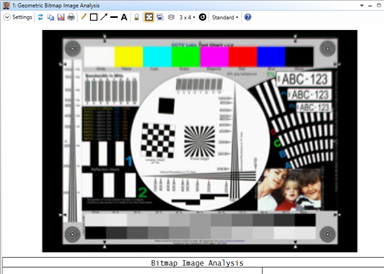The MTF plot for this lens is shown below. It shows the contrast ratio for any spatial frequency up to the maximum that the lens can support. In this case, we choose to limit the spatial frequencies shown to a maximum of 100 cycles/mm. Also shown for reference is the diffraction-limited performance of an aberration-free lens of the same f/#.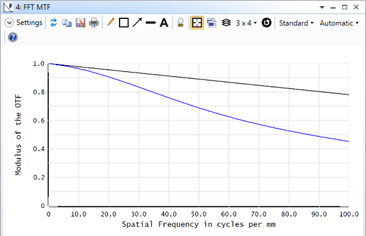Note: The apparent image quality is reduced by screen resolution and the compression of the image. The original image as produced by OpticStudio is of photographic quality.

Fraunhofer (FFT) MTF

The MTF algorithm used by the above analysis feature, which produces a graph of MTF for all spatial frequencies that the lens can support, is based upon the Fraunhofer diffraction theory. The method involves a Fast Fourier Transform of a grid of rays traced to the pupil (hence the name FFT MTF). The resulting MTF is the modulation as a function of spatial frequency for a sine wave object; although optionally the real, imaginary, phase, and square wave response is available.

FFT-based methods of computing the Point Spread Function and MTF are well known and are based on Fraunhofer diffraction theory. The primary assumptions made are:

• The f/# is large enough so that scalar diffraction theory applies

• The region over which the diffraction PSF has significant energy is small compared to the distance from the exit pupil of the optical system to the image surface

• The exit pupil is not significantly distorted concerning the entrance pupil. This means uniform distribution of rays on the entrance pupil remains reasonably uniform on the exit pupil

• The sampling is set high enough to accurately model the PSF

Many - but not all-optical imaging systems meet the simplifying assumptions necessary for the Fraunhofer diffraction theory used by the FFT MTF algorithm.

When it comes to optimization, usually only specific spatial frequencies are required, and it is unnecessary to compute the MTF at all supported frequencies. Therefore, the MTF* operands that compute MTF at a specific spatial frequency support a grid parameter that can switch between the grid method used by the graphics window and a fast, sparse-sampling method that is the default and strongly recommended for most (almost all) optimization cases. This is discussed in more detail in the Help System file The Optimize Tab (sequential UI mode)>Automatic Optimization Group>Merit Function Editor (automatic optimization group>Optimization Operands (Alphabetically).

In a manner like Gaussian Quadrature, the sparse sampling computation converges very, very quickly, and computes the MTF to arbitrary precision with vastly fewer rays than the grid method. And, most importantly, it is fully accurate in all cases where the Fraunhofer theory is applicable.

The following table demonstrates the convergence of the two methods. The table records the time taken to converge as the sampling is increased for a calculation of the polychromatic MTF at 50 lp/mm (on-axis) in the double Gauss sample file provided with OpticStudio: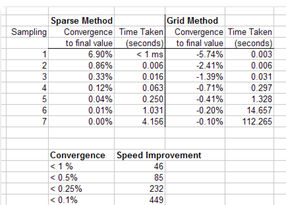Here is the same data, this time for the edge of the field of view:Note that generally speaking, 1% convergence is adequate for optimization and tolerancing. Experimental methods of measuring MTF are generally not repeatable below 0.1% in any case. Extreme precision is not required for good optimization results; three significant figures are usually adequate. However, both algorithms will converge to arbitrary precision with adequate sampling, and the fast algorithm will do so many orders of magnitude faster when extreme precision is needed.

The grid algorithm will converge faster in only one known case: where aberrations are very large and the resulting MTF very low: less than about 5%. Note that MTF is not normally used to specify performance, much less be used as an optimization or tolerancing target, in this regime. OpticStudio automatically traps this condition and switches to the grid method in this case. Note also that the geometric MTF calculation is a better choice in this regime.

Geometric MTF

Imagine using a 35-mm SLR camera lens at full aperture with an f/# of f/1.8. Here, the lens aberrations are most severe at full aperture. As the lens is stopped down, aberrations are reduced, and the image quality is improved. However, the improvement in image quality does not last forever; in some settings of the aperture, diffraction from the aperture prevents you from gaining any further improvement in image quality.

For cases where there are many waves of aberration, the Geometric MTF calculation and GMT* optimization operands are recommended. It is based on a Fourier transform of the geometric spot data and uses a Gaussian Quadrature sampling scheme.

The primary advantage to using the geometric MTF is very high speed compared to the diffraction calculation. The geometric MTF is very accurate for systems with large aberrations, where the diffraction MTF calculation would need enormous sampling to converge. The geometric calculation is typically factors of 100 or faster in this regime.

The Geometric MTF calculation can even account for scattering from optical surfaces, which reduces MTF by increasing the background illumination. Additionally, the Geometric MTF is very useful in Global Search, where one wants to efficiently search all parameter space to identify regions where good designs can be found.

Huygens MTF

The Huygens MTF is not based upon the FFT. With this method, the only assumptions are that the f/# is large enough so that scalar diffraction theory applies and that the sampling is set high enough to accurately model the PSF. You can read more about the Huygens calculation in the article, "What is a Point Spread Function."

Virtually all imaging systems meet the simplifying assumptions necessary for computing the Huygens PSF. The Huygens MTF is generally slower than the FFT MTF, but more accurate for those cases where the FFT MTF assumptions do not apply.

There is another case where only the Huygens’s calculation can be used: if Chief Ray cannot be traced through the system, then a Chief Ray-centered reference sphere cannot be created. The reference sphere is essential for many wavefront calculations. In this case, the Huygens PSF and MTF calculations can be used. One such case is this multiple mirror telescope, in which Chief Ray has no path to the image surface.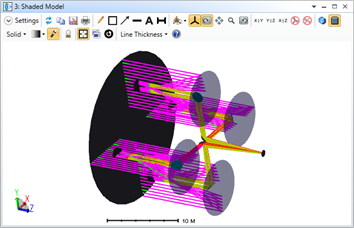Because Chief Ray cannot trace to the image surface, OPD cannot be determined, and any parameter derived from OPD cannot be calculated:The Huygens' PSF and MTF calculations work perfectly, as they are not dependent on anyone ray used as a reference: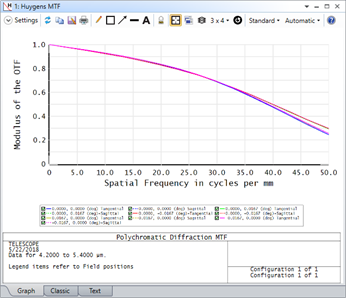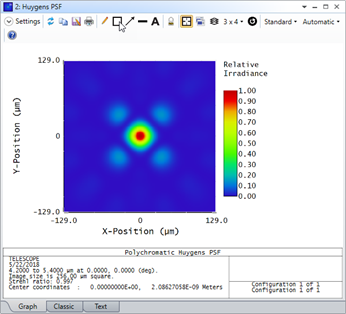The above example file can be found in the Zemax install folder at the following location: {Zemax}\Samples\Non-sequential\Miscellaneous\Multiple mirror telescope.ZOS.

A final benefit of the Huygens method is that the MTFs over multiple configurations can be summed. This is of use in multiple mirror telescopes, particularly in systems with very long baseline instruments, where the primary mirrors are separated by large distances. In this case, it is not desired to use a common entrance pupil, because too few rays hit the primary mirrors to be efficient. Thus, the Huygens MTF calculation is uniquely capable.

To optimize or tolerance using Huygens, MTF, use the MTH* operands.

Method comparison

• Fraunhofer-theory-based FFT methods are the most common approach. OpticStudio computes the MTF for all spatial frequencies supported by a lens (though it will optionally only display those frequencies of interest) in a graph of MTF versus frequency. When optimizing or tolerancing on MTF, only the spatial frequency of interest is computed, which is much faster and requires far fewer rays for a given level of precision. The grid method of computing MTF is available as an option if required.

• For conventional optical systems with large aberrations the Geometric MTF computes an approximate MTF with great speed. It is a good choice for "roughing it" a design for best MTF as fast as one can optimize RMS spot radius.

• For systems that do not meet the assumptions of Fraunhofer theory, or in which Chief Ray cannot be traced, the Huygens' calculation is available. This provides a robust calculation of MTF. The only disadvantage of the Huygens method is speed.

To learn more on how to use Contrast Optimization to optimize for MTF performance check out the additional resources below: